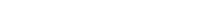# Math riddles Level 59 Answer with Hints and solutionsMath riddles game level 59 Answer with solution android game developed by Black games. Scroll Below to find details.

Math Riddles tests your IQ with mathematical puzzles. Challenge yourself with different levels of math puzzles and stretch the limits of your intelligence. Every IQ game is prepared with an approach of an IQ test. Improve your mathematics, challenge your brain with this game. There are many hard levels which you may not have solved then you can take help from here.

Math app level 59:Solution: the sum of two boxes /2, Like

(7+5)/2=6

(11+3)/2=7

(20+2)/2=11

similarly

(4+10)/2=7

If there is any Doubt/discrepancy then please let me know in comments or you are unable to understand solution then I will surely help you.

### 6 thoughts on “Math Riddles App Level 59 Solution Android”

•October 30, 2019 at 2:36 am

But howwwwww.. I still can’t understand

•July 4, 2019 at 4:17 pm

I summed 3 L shaped numbers, then found out that the result is the top number x3

This is not a riddle is pure stupidity

•September 10, 2019 at 6:20 am

You still get 7 though, right?

•April 21, 2019 at 1:52 am

•April 3, 2019 at 7:39 pm
•